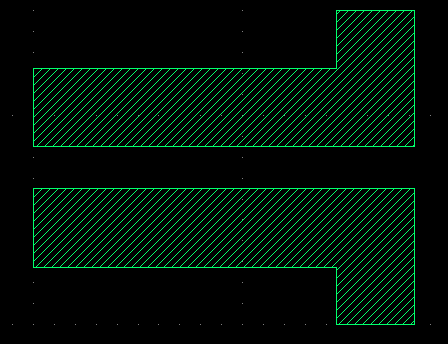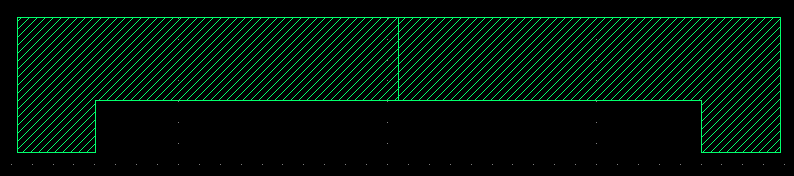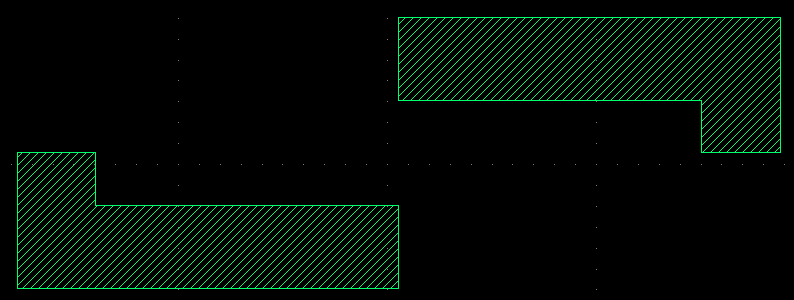# Region transformed with respect to the specific axis

edited July 5

Hello,

I need to make the cell mirrored with respect to the x axis.
I tried two different ways,
one is that, 180 degree rotate, and mirrored. And the other is that, 180 degree rotate without mirror, and then mirror it with two lines.

This is the first trial

``````nSource_lower = ...
nSource_upper = nSource_lower.transformed(RBA::ICplxTrans::new(1, 180, true, 0, SOMEDOUBLE))
nSource_box = nSource_lower + nSource_upper
``````

and this is the second

``````nSource_lower = ...
nSource_upper = nSource_lower.transformed(RBA::ICplxTrans::new(1, 180, false, 0, SOMEDOUBLE))
nSource_upper = nSource_lower.transformed(RBA::ICplxTrans::new(1, 0, true, 0, SOMEDOUBLE))
nSource_box = nSource_lower + nSource_upper
``````

For the first trial, it rotated with respect to the y axis, but for the second trial, it rotated w.r.t. the x axis.

This is the final cell that I want to make.
1)This nSource_box is from the first trial
2)And this is the second.
3)4)image 3) is just rotated cell, and image 4) is mirrored.

I want to move the transformed cell by (0, SOMEDOUBLE) from the original cell.
But apparently, it does not move any direction.

If I have a box below, how to make the final goal by using transformed the lower cell?
I think it would be the most simple that, if I can mirror the lower cell and move it to the proper location.

Would you give me recommendation?

Thank you very much